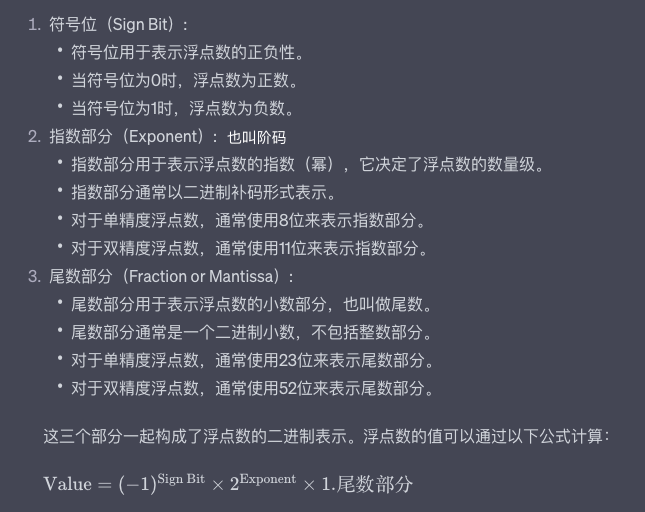# Encode¶

ASCII 字符表前32个字符都是控制字符，很多都源于遥远的电报时代

Unicode只规定了每个字符对应的码点（数字），而一个码点占用几个字节由Unicode的编码方式UTF规定，也叫字符集，比如UTF-32，规定四个字节表示一个码点，但这出现了一个问题，就是如果文本都是纯英文的话，那么就比直接用ASCII多4倍的存储空间。所以出现了边长型的UTF-8编码，它会根据不同码点大小占用1~6个字节，比如英语通常为1Byte，汉字为3Byte，很节省空间。一般在计算机内存中会统一使用Unicode，但在存储和传输的时候都会转换为UTF-8以节省空间和提高性能。

Python3.x默认使用UTF-8，但像Python2.x等编程语言比 Unicode 诞生要早，所以默认编码是ASCII，所以一般需要在源码开头声明编码格式

``````#!/usr/bin/python
# -*- coding: utf-8 -*-
"""

"""
``````

## 进制转换¶

• 十进制(Decimal)：人类有十个手指头，为了方便理解，通常使用十进制
• 二进制(Binary)：计算机芯片内使用的是门电路，只能表示0和1两个状态，所以使用二进制
• 八进制(Octal)

### 与十进制互转¶

• 10进制转任意进制：除基取余，直到商为0，余数倒序
``````bin(15)  # 转成二进制 0b1111
oct(15)  # 转成八进制 0o17
hex(15)  # 转成十六进制 0xf
``````
• 任意进制转10进制：系数*基数^权次幂（幂从0开始）
``````"""

0,1,2,3,4,5,6,7,8,9,10,11,12,13,14,15...
1024 = 1*10^3+0*10^2+2*10^1+4*10^0 = 1*1000+0*100+2*10+4*1 = 1024

0,1,10,11,100,101,110,111...
0b110 = 1*2^2+1*2^1+0*2^0 = 1*4+1*2+0*1 = 6

0,1,2,3,4,5,6,7,10,11,12,13,14,15...
0o711 = 7*8^2+1*8^1+1*8^0 = 7*64+1*8+1*1 = 457

0,1,2,3,4,5,6,7,8,9,A,B,C,D,E,F,10,11...
0xABC = 10*16^2+11*16^1+12*16^0 = 10*256+11*16+12*1 = 2748

"""

int(0b1111)  # 15
int(0o17)  # 15
int(0xf)  # 15
``````

### 与二进制互转¶

• 2进制与8进制互转：3位一组，421码
``````oct(0b1101)  # 0o15
bin(0o15)  # 0b1101
``````
• 2进制与16进制互转：4位一组，8421码
``````hex(0b1101)  # 0xd
bin(0xd)  # 0b1101
``````

## 浮点数存储¶``````比如 13.75

``````

``````比如 0.1

``````